## Magnetostatics, Theory

Electromagnetics   Magnetostatics

### Magnetostatics in Free Space

Magnetostatics is the subfield of electromagnetics describing a static magnetic field, such as the one generated by a steady electric current or a permanent magnet. Starting with free space, the equations of magnetostatics are Gauss's magnetic law:

(1)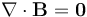and Maxwell–Ampère's law (static version):

(2)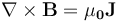where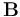is the magnetic flux density,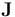is the current density, and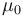is the permeability of vacuum.

Note that the magnetic version of Gauss's law implies that there are no magnetic charges. A further consequence of this law is that the magnetic flux density is solenoidal, or divergence free. This means that the field can be written as the curl of another vector field as follows:

(3)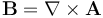where the field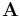is called the magnetic vector potential.

The electric potential allows for a more efficient way of expression for the equations of electrostatics and steady currents. In a similar way, the magnetic vector potential allows for a more efficient way of formulating the equations of magnetostatics, as shown further below.

Helmholtz's theorem says that a vector field is defined (up to a constant) by its curl and divergence. The choice of divergence of the magnetic vector potential is nontrivial. One of several choices is the Coulomb gauge:

(4)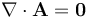Using the magnetic vector potential, the equations of magnetostatics in free space can be combined into one equation:

(5)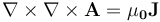The vector identity:

(6)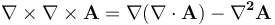together with the Coulomb gauge condition gives another version of the magnetostatics equation in free space:

(7)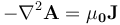#### Magnetostatics in Magnetic Materials

A magnetic material is characterized by having a permanent or induced magnetic moment. Because of this, the magnetic flux density inside a magnetic material will be different from that of free space.

To get a macroscopic description of this phenomenon, it is convenient to introduce a magnetization vector field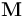, and a magnetic field intensity,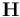. They are related by:

(8)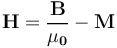whereis the magnetic permeability.

This relationship is analogous to:

(9)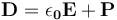where, for historical reasons, in the magnetic case, the coefficient of proportionality occurs as a reciprocal, and the magnetization field has a negative sign.

The magnetization vector field can be seen as generating an equivalent volume current density,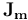, according to:

(10)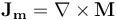and the magnetostatics equation in free space can be generalized to include material effects as follows:

(11)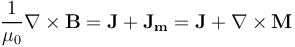The magnetic field intensity then allows the magnetostatics equation to be written as:

(12)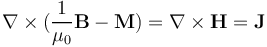whereis the free current density.

Since the magnetic vector potential encodes the fact that the magnetic flux density is divergence free, the equations of magnetostatics can be combined into a single equation

(13)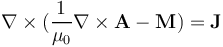#### Linear Magnetic Materials

For a linear magnetic material, the magnetization is proportional to the magnetic field intensity: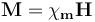where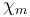is the magnetic susceptibility.

The relationship with the magnetic flux density is: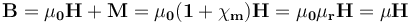where two new useful quantities are introduced: the relative permeability,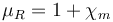, and the absolute permeability,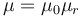.

Based on this, the fundamental equation for magnetostatics in linear isotropic materials is: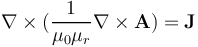In the case of an anisotropic material, the relative magnetic susceptibility and permeability can be three-by-three tensors. In the case of permeability:

(14)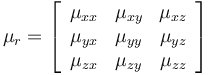Because of the reciprocal relationship of the permeability, the equation of magnetostatics for the anisotropic case is:

(15)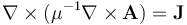where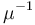is the inverse of the permeability tensor,.

#### Magnetostatics Equations and Boundary Conditions at Material Interfaces

The most important equations in magnetostatics are summarized in the following table:

Equation Name Differential Form Integral Form Boundary Condition
Gauss's magnetic law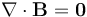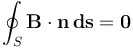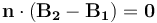Maxwell–Ampère's law (magnetostatics)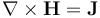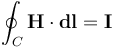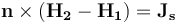where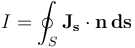is the current through the closed contour C and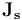is the surface current density.

The meaning of Faraday's law in the theory of steady currents is identical to that of electrostatics. The meaning of the current conservation equations can be summarized in words as follows.

Equation Name Differential Form Integral Form Boundary Condition
Gauss's magnetic law There are no magnetic charges. The magnetic flux is conserved. The magnetic flux lines always close upon themselves. The normal component of the magnetic flux density is continuous.
Maxwell–Ampère's law (magnetostatics) The curl (infinitesimal circulation) of the magnetic field at a point equals the current density at that point. The circulation of the magnetic field around a closed path equals the current flowing through the surface bounded by the path. The surface current at a material interface equals the jump in the tangential component of the magnetic field.A spiral inductor carrying a steady current. The flux lines corresponding to the magnetic flux density,, are visualized in the surrounding air. The flux lines are color coded according to the magnitude of the flux. Blue and red represent low and high magnitude values, respectively.

A spiral inductor carrying a steady current. The flux lines corresponding to the magnetic flux density,, are visualized in the surrounding air. The flux lines are color coded according to the magnitude of the flux. Blue and red represent low and high magnitude values, respectively.The magnitude of the magnetic flux density,, on a plane through the main structure of a spiral inductor. Red and white represent low and high magnitude values, respectively.

The magnitude of the magnetic flux density,, on a plane through the main structure of a spiral inductor. Red and white represent low and high magnitude values, respectively.

### Magnetostatics with No Free Currents

For a situation where there are no free currents, but only a magnetization vector field, Maxwell–Ampère's law takes the simplified form: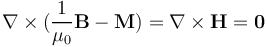The fact that the magnetic intensity field is irrotational (curl free) means that a scalar potential exists (say,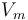), such that: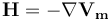This can be combined with Gauss's magnetic law and: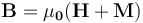to get the following equation for magnetostatics with no free currents: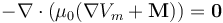This equation is analogous to the equation of electrostatics and can be used, for example, to model permanent magnets.

The left image displays the magnetic flux density,, around a permanent horseshoe magnet and an iron rod. The arrows show the directions of the magnetic flux density, and the color of the intersecting plane shows the magnitude of the flux. Pink and blue represent a low and high value of the flux magnitude, respectively. The right image shows the corresponding geometry.

#### Linear Magnetic Materials

For linear magnetic materials, the equation for magnetostatics with no free currents becomes: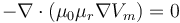#### Magnetostatic Energy

The magnetostatic energy contained in a field can be expressed in many different ways; for example, depending on if the material is linear or not. For a linear material, the magnetostatic energy in a volume,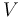, expressed in field quantities is: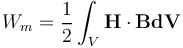where the magnetostatic energy density is defined as: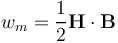An alternative expression of magnetostatic energy in terms of the magnetic vector potential and the current density is: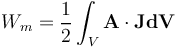and the two expressions for energy can be shown to be equivalent.

For nonlinear materials, a more complicated expression is needed, since the history of the "magnetic loading" of the material is important.

The concept of magnetostatic energy is useful when computing electrostatic forces and inductance values.

Published: February 26, 2019